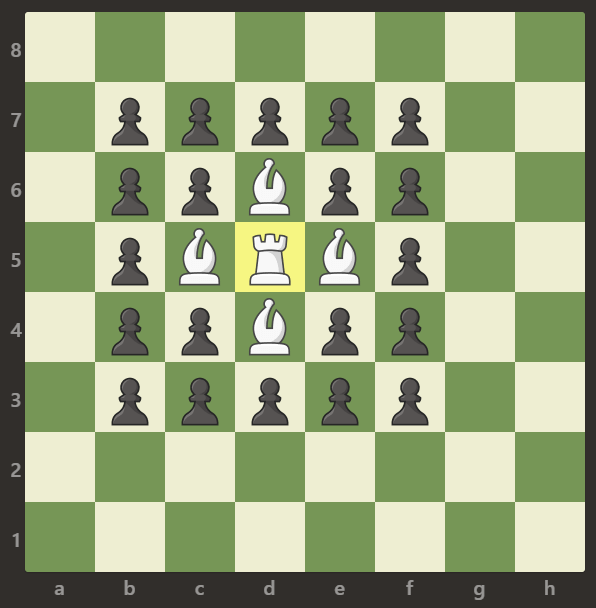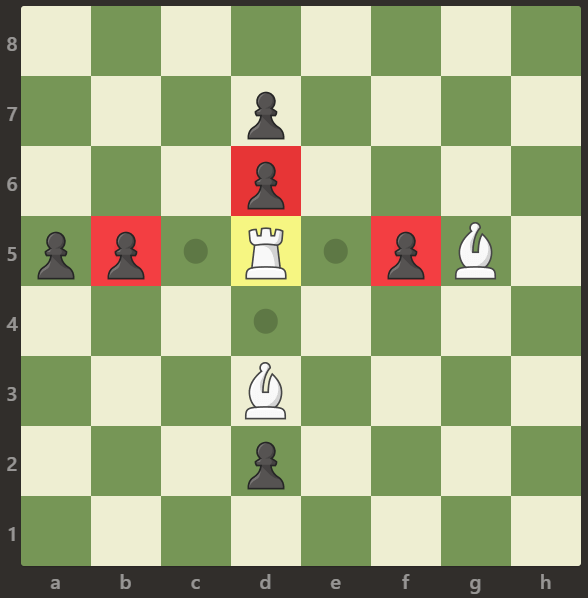999.Available Captures for Rook
999.Available Captures for Rook

1

2

3

4

Copied!1

2

3

4

Copied!1

2

3

4

5
6

7
board.length == board[i].length == 8
8
board[i][j] 可以是 'R'，'.'，'B' 或 'p'
9

Copied!

1
class Solution {
2
public:
3
int numRookCaptures(vector<vector<char>>& board) {
4
vector<int> R={0,0};
5
for(int i=0;i<8;i++)
6
for(int j=0;j<8;j++)
7
if(board[i][j]=='R')
8
{
9
R=i;
10
R=j;
11
break;
12
}
13
int cnt=0;
14
for(int k=R;k<8;k++)
15
if(board[R][k]=='p'){cnt++;break;}
16
else if(board[R][k]=='B') break;
17
for(int k=R;k<8;k++)
18
if(board[k][R]=='p'){cnt++;break;}
19
else if(board[k][R]=='B') break;
20
for(int k=R;k>=0;k--)
21
if(board[R][k]=='p') {cnt++;break;}
22
else if(board[R][k]=='B') {break;}
23
for(int k=R;k>=0;k--)
24
if(board[k][R]=='p'){cnt++;break;}
25
else if(board[k][R]=='B') break;
26
return cnt;
27
28
}
29
};
Copied!